# The dough

The dough contains water, flour, and sugar. Water and flour in a ratio of 2: 3, flour and sugar in a 2: 1. Find the ratio of all three components of the dough.

Result

r = (Correct answer is: 4:6:3)### Step-by-step explanation: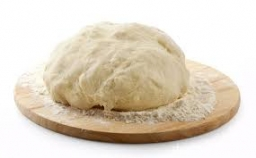Did you find an error or inaccuracy? Feel free to write us. Thank you!Tips to related online calculators
Need help to calculate sum, simplify or multiply fractions? Try our fraction calculator.
Check out our ratio calculator.

## Related math problems and questions:

• The dough 2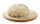A baker made 8 pounds of dough. He used 5/9 of the dough to make sandwich rolls. How much of the dough is left over?
• Nutballs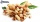The dough for nutballs contains, among other things, two basic raw materials: flour and nuts in a ratio of 2:1. How much is flour, and how many nuts are needed for 1 kg of dough if "other" is 100g?
• The angles ratioThe angles in the ABC triangle are in the ratio 1: 2: 3. find the sizes of the angles and determine what kind of a triangle it is.
• Baker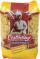The baker makes from 10 kg of flour 12 kg dough. How much flour he need to make a 100 kg dough?
• Cake ingredients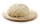The yeast cake contains milk, yeast, sugar, and flour in a ratio of 20: 4: 1: 15. What percentage of flour is in yeast?
• Find unknown 2Find unknown denominator: 2/3 -5/? = 1/4
• FlourKim needs 3/4 cup of flour to make 12 cookies. How much flour would she need to make 60 cookies?
• Unknown number 5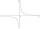Daniel thinks an integer. When he change this number at a ratio of 2:5, he got the number 2.8. Determine what number think Daniel.
• Sugar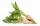From 3 tons of sugar beet was produced 480 kg of sugar. How many tons of sugar was produced from 17.5 tons of sugar beet?
• PumpsPump that draws water at velocity 3.5 liters per second water from a construction trench take 35 minutes. a) Find out how many minutes the water would run out of the trench pump that draws 7.4 liters of water per second. b) What is the pumping velocity wo
• Evaluate expr with 2 varsIf a=6 and x=2, find the value of (2ax + 7x- 10) /(4ax-3a-2)
• Sayavong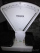Sayavong is making cookies for the class. He has a recipe that calls for 3 and 1/2 cups of flour. He has 7/8 of a cup of wheat flour, and 2 and 1/2 cups of white flour. Does Mr. Sayavong have enough flour to make the cookies?
• Recipe 2Anna's making muffins! These ingredients make 12 muffins: 3/4 cup of flour 1/2 cup of sugar 1/4 teaspoon salt 1 cup of milk 1/3 teaspoon cinnamon 7 tablespoons butter 2 teaspoons baking powder 1 egg 2 tablespoons brown sugar Anna has a 8 muffin tray. What
• When 5When 5 is subtracted from both my numerator and denominator I become 3/4. What fraction i am?
• Sugar production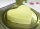From 1 ton of beet, 150 kg of sugar is produced. To clean 1 ton of sugar, 450 kg of lime is consumed. Calculate how many kgs of lime is consumed when processing 1 ton of sugar beet?
• Two thirdsFind two-thirds of the number equal to two-thirds of 99
• Ratio of volumesIf the heights of two cylindrical drums are in the ratio 7:8 and their base radii are in the ratio 4:3. What is the ratio of their volumes?